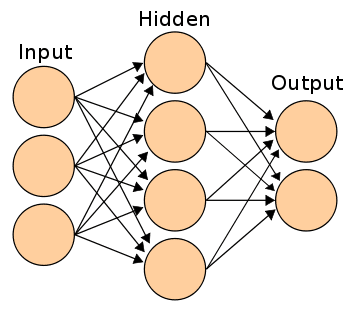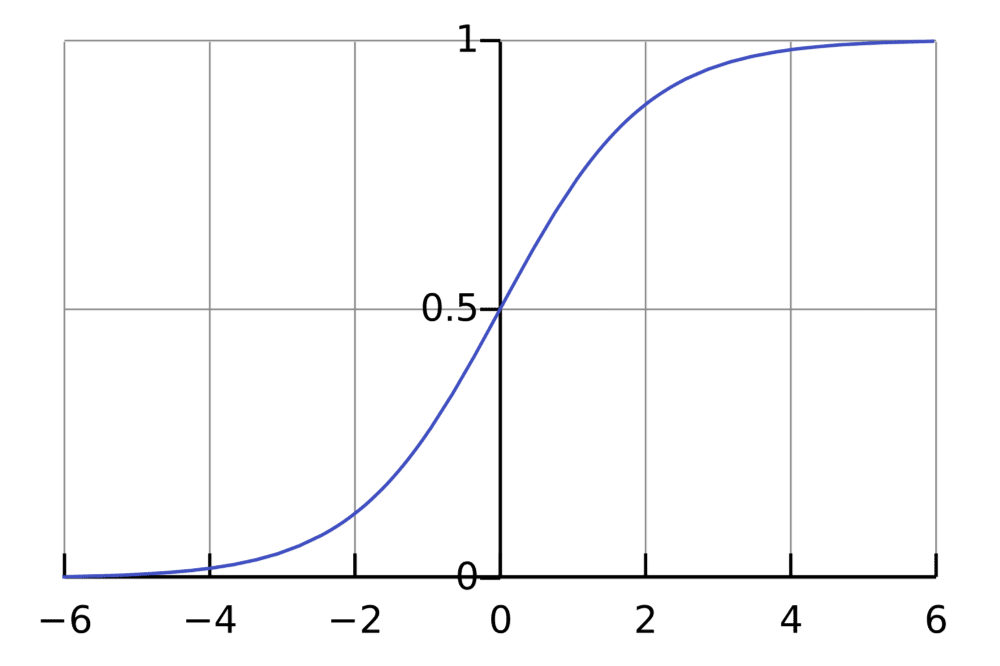# Learning to Code Neural Networks

Learn how to code a neural network, by taking advantage of someone else's experiences learning how to code a neural network.

By Per Harald Borgen, Xeneta.

This is the second post in a series of me trying to learn something new over a short period of time. The first time consisted of learning how to do machine learning in a week.

This time I’ve tried to learn neural networks. While I didn’t manage to do it within a week, due to various reasons, I did get a basic understanding of it throughout the summer and autumn of 2015.

By basic understanding, I mean that I finally know how to code simple neural networks from scratch on my own.

In this post, I’ll give a few explanations and guide you to the resources I’ve used, in case you’re interested in doing this yourself.

Step 1: Neurons and forward propagation

So what is a neural network? Let’s wait with the network part and start off with one single neuron.

A neuron is like a function; it takes a few inputs and calculates an output.

The circle below illustrates an artificial neuron. Its input is 5 and its output is 1. The input is the sum of the three synapses connecting to the neuron (the three arrows at the left).At the far left we see two input values plus a bias value. The input values are 1 and 0 (the green numbers), while the bias holds a value of -2 (the brown number).

The inputs here might be numerical representations of two different features. If we’re building a spam filter, it could be whether or not the email contains more than one CAPITALIZED WORD and whether or not it contains the word ‘viagra’.

The two inputs are then multiplied by their so called weights, which are 7 and 3 (the blue numbers).

Finally we add it up with the bias and end up with a number, in this case: 5 (the red number). This is the input for our artificial neuron.The neuron then performs some kind of computation on this number — in our case the Sigmoid function, and then spits out an output. This happens to be 1, as Sigmoid of 5 equals to 1, if we round the number up (more info on the Sigmoid function follows later).

If this was a spam filter, the fact that we’re outputting 1 (as opposed to 0) probably means that the neuron has labeled the text as ‘spam’.A neural network illustration from Wikipedia.

If you connect a network of these neurons together, you have a neural network, which propagates forward — from input output, via neurons which are connected to each other through synapses, like on the image to the left.

I can strongly recommend the Welch Labs videos on YouTube for getting a better intuitive explanation of this process.

Step 2: Understanding the Sigmoid function

After you’ve seen the Welch Labs videos, its a good idea to spend some time watching Week 4 of the Coursera’s Machine Learning course, which covers neural networks, as it’ll give you more intuition of how they work.

The course is fairly mathematical, and its based around Octave, while I prefer Python. Because of this, I did not do the programming exercises. Instead, I used the videos to help me understand what I needed to learn.

The first thing I realized I needed to investigate further was the Sigmoid function, as this seemed to be a critical part of many neural networks. I knew a little bit about the function, as it was also covered in Week 3 of the same course. So I went back and watched these videos again.The Sigmoid function simply maps your value (along the horizontal axis) to a value between 0 and 1.

But watching videos won’t get you all the way. To really understand it, I felt I needed to code it from the ground up.

So I started to code a logistic regression algorithm from scratch (which happened to use the Sigmoid function).

It took a whole day, and it’s probably not a very good implementation of logistic regression. But that doesn’t matter, as I finally understood how it works. Check the code here.

You don’t need to perform this entire exercise yourself, as it requires some knowledge about and cost functions and gradient descent, which you might not have at this point.

But make sure you understand how the Sigmoid function works.